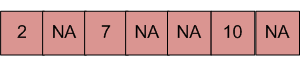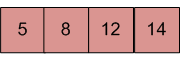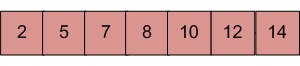Related Articles

# Merge an array of size n into another array of size m+n

• Difficulty Level : Easy
• Last Updated : 22 Mar, 2021

There are two sorted arrays. First one is of size m+n containing only m elements. Another one is of size n and contains n elements. Merge these two arrays into the first array of size m+n such that the output is sorted.
Input: array with m+n elements (mPlusN[]).NA => Value is not filled/available in array mPlusN[]. There should be n such array blocks.
Input: array with n elements (N[]).Output: N[] merged into mPlusN[] (Modified mPlusN[])Algorithm:

```Let first array be mPlusN[] and other array be N[]
1) Move m elements of mPlusN[] to end.
2) Start from nth element of mPlusN[] and 0th
element of N[] and merge them into mPlusN[].```

Below is the implementation of the above algorithm :

## C++

 `// C++ program to Merge an array of``// size n into another array of size m + n``#include ``using` `namespace` `std;` `/* Assuming -1 is filled for the places``   ``where element is not available */``#define NA -1` `/* Function to move m elements at``   ``the end of array mPlusN[] */``void` `moveToEnd(``int` `mPlusN[], ``int` `size)``{``   ``int` `j = size - 1;``   ``for` `(``int` `i = size - 1; i >= 0; i--)``     ``if` `(mPlusN[i] != NA)``     ``{``        ``mPlusN[j] = mPlusN[i];``        ``j--;``     ``}``}` `/* Merges array N[] of size n into``   ``array mPlusN[] of size m+n*/``int` `merge(``int` `mPlusN[], ``int` `N[], ``int` `m, ``int` `n)``{``   ``int` `i = n; ``/* Current index of i/p part of mPlusN[]*/``   ``int` `j = 0; ``/* Current index of N[]*/``   ``int` `k = 0; ``/* Current index of output mPlusN[]*/``   ``while` `(k < (m + n))``   ``{``    ``/* Take an element from mPlusN[] if``    ``a) value of the picked element is smaller``       ``and we have not reached end of it``    ``b) We have reached end of N[] */``    ``if` `((j == n)||(i < (m + n) && mPlusN[i] <= N[j]))``    ``{``        ``mPlusN[k] = mPlusN[i];``        ``k++;``        ``i++;``    ``}``    ``else` `// Otherwise take element from N[]``    ``{``       ``mPlusN[k] = N[j];``       ``k++;``       ``j++;``    ``}``   ``}``}` `/* Utility that prints out an array on a line */``void` `printArray(``int` `arr[], ``int` `size)``{``   ``for` `(``int` `i = 0; i < size; i++)``   ``cout << arr[i] << ``" "``;` `   ``cout << endl;``}` `/* Driver code */``int` `main()``{``   ``/* Initialize arrays */``   ``int` `mPlusN[] = {2, 8, NA, NA, NA, 13, NA, 15, 20};``   ``int` `N[] = {5, 7, 9, 25};``   ` `   ``int` `n = ``sizeof``(N) / ``sizeof``(N);``   ``int` `m = ``sizeof``(mPlusN) / ``sizeof``(mPlusN) - n;` `   ``/*Move the m elements at the end of mPlusN*/``   ``moveToEnd(mPlusN, m + n);` `   ``/*Merge N[] into mPlusN[] */``   ``merge(mPlusN, N, m, n);` `   ``/* Print the resultant mPlusN */``   ``printArray(mPlusN, m+n);` `   ``return` `0;``}`

## C

 `// C program to Merge an array of``// size n into another array of size m + n``#include ` `/* Assuming -1 is filled for``   ``the places where element``   ``is not available */``#define NA -1` `/* Function to move m elements``   ``at the end of array mPlusN[]`` ``*/``void` `moveToEnd(``int` `mPlusN[], ``int` `size)``{``    ``int` `i = 0, j = size - 1;``    ``for` `(i = size - 1; i >= 0; i--)``        ``if` `(mPlusN[i] != NA) {``            ``mPlusN[j] = mPlusN[i];``            ``j--;``        ``}``}` `/* Merges array N[] of size n into array mPlusN[]``   ``of size m+n*/``int` `merge(``int` `mPlusN[], ``int` `N[], ``int` `m, ``int` `n)``{``    ``int` `i = n; ``/* Current index of i/p part of mPlusN[]*/``    ``int` `j = 0; ``/* Current index of N[]*/``    ``int` `k = 0; ``/* Current index of output mPlusN[]*/``    ``while` `(k < (m + n))``    ``{``        ``/* Take an element from mPlusN[] if``           ``a) value of the picked element is smaller and we``           ``have not reached end of it``           ``b) We have reached end of N[] */``        ``if` `((j == n)|| (i < (m + n)``             ``&& mPlusN[i] <= N[j]))``        ``{``            ``mPlusN[k] = mPlusN[i];``            ``k++;``            ``i++;``        ``}``        ``else` `// Otherwise take element from N[]``        ``{``            ``mPlusN[k] = N[j];``            ``k++;``            ``j++;``        ``}``    ``}``}` `/* Utility that prints out an array on a line */``void` `printArray(``int` `arr[], ``int` `size)``{``    ``int` `i;``    ``for` `(i = 0; i < size; i++)``        ``printf``(``"%d "``, arr[i]);` `    ``printf``(``"\n"``);``}` `/* Driver code */``int` `main()``{``    ``/* Initialize arrays */``    ``int` `mPlusN[] = { 2, 8, NA, NA, NA, 13, NA, 15, 20 };``    ``int` `N[] = { 5, 7, 9, 25 };``    ``int` `n = ``sizeof``(N) / ``sizeof``(N);``    ``int` `m = ``sizeof``(mPlusN) / ``sizeof``(mPlusN) - n;` `    ``/*Move the m elements at the end of mPlusN*/``    ``moveToEnd(mPlusN, m + n);` `    ``/*Merge N[] into mPlusN[] */``    ``merge(mPlusN, N, m, n);` `    ``/* Print the resultant mPlusN */``    ``printArray(mPlusN, m + n);` `    ``return` `0;``}`

## Java

 `// Java program to Merge an array of``// size n into another array of size m + n` `class` `MergeArrays {``    ``/* Function to move m``       ``elements at the end of array``     ``* mPlusN[] */``    ``void` `moveToEnd(``int` `mPlusN[], ``int` `size)``    ``{``        ``int` `i, j = size - ``1``;``        ``for` `(i = size - ``1``; i >= ``0``; i--) {``            ``if` `(mPlusN[i] != -``1``) {``                ``mPlusN[j] = mPlusN[i];``                ``j--;``            ``}``        ``}``    ``}` `    ``/* Merges array N[] of``       ``size n into array mPlusN[]``       ``of size m+n*/``    ``void` `merge(``int` `mPlusN[], ``int` `N[], ``int` `m, ``int` `n)``    ``{``        ``int` `i = n;` `        ``/* Current index of i/p part of mPlusN[]*/``        ``int` `j = ``0``;` `        ``/* Current index of N[]*/``        ``int` `k = ``0``;` `        ``/* Current index of output mPlusN[]*/``        ``while` `(k < (m + n))``        ``{``            ``/* Take an element from mPlusN[] if``            ``a) value of the picked element is smaller and we``            ``have not reached end of it b) We have reached``            ``end of N[] */``            ``if` `((i < (m + n) && mPlusN[i] <= N[j])``                ``|| (j == n)) {``                ``mPlusN[k] = mPlusN[i];``                ``k++;``                ``i++;``            ``}``            ``else` `// Otherwise take element from N[]``            ``{``                ``mPlusN[k] = N[j];``                ``k++;``                ``j++;``            ``}``        ``}``    ``}` `    ``/* Utility that prints out an array on a line */``    ``void` `printArray(``int` `arr[], ``int` `size)``    ``{``        ``int` `i;``        ``for` `(i = ``0``; i < size; i++)``            ``System.out.print(arr[i] + ``" "``);` `        ``System.out.println(``""``);``    ``}` `    ``// Driver Code``    ``public` `static` `void` `main(String[] args)``    ``{``        ``MergeArrays mergearray = ``new` `MergeArrays();` `        ``/* Initialize arrays */``        ``int` `mPlusN[] = { ``2``, ``8``, -``1``, -``1``, -``1``, ``13``, -``1``, ``15``, ``20` `};``        ``int` `N[] = { ``5``, ``7``, ``9``, ``25` `};``        ``int` `n = N.length;``        ``int` `m = mPlusN.length - n;` `        ``/*Move the m elements at the end of mPlusN*/``        ``mergearray.moveToEnd(mPlusN, m + n);` `        ``/*Merge N[] into mPlusN[] */``        ``mergearray.merge(mPlusN, N, m, n);` `        ``/* Print the resultant mPlusN */``        ``mergearray.printArray(mPlusN, m + n);``    ``}``}` `// This code has been contributed by Mayank Jaiswal`

## Python3

 `# Python program to Merge an array of``# size n into another array of size m + n` `NA ``=` `-``1` `# Function to move m elements``# at the end of array mPlusN[]`  `def` `moveToEnd(mPlusN, size):` `    ``i ``=` `0``    ``j ``=` `size ``-` `1``    ``for` `i ``in` `range``(size``-``1``, ``-``1``, ``-``1``):``        ``if` `(mPlusN[i] !``=` `NA):` `            ``mPlusN[j] ``=` `mPlusN[i]``            ``j ``-``=` `1` `# Merges array N[]``# of size n into array mPlusN[]``# of size m+n`  `def` `merge(mPlusN, N, m, n):` `    ``i ``=` `n  ``# Current index of i/p part of mPlusN[]``    ``j ``=` `0`  `# Current index of N[]``    ``k ``=` `0`  `# Current index of output mPlusN[]``    ``while` `(k < (m``+``n)):` `        ``# Take an element from mPlusN[] if``        ``# a) value of the picked``        ``# element is smaller and we have``        ``# not reached end of it``        ``# b) We have reached end of N[] */``        ``if` `((j ``=``=` `n) ``or` `(i < (m``+``n) ``and` `mPlusN[i] <``=` `N[j])):` `            ``mPlusN[k] ``=` `mPlusN[i]``            ``k ``+``=` `1``            ``i ``+``=` `1` `        ``else``:  ``# Otherwise take element from N[]` `            ``mPlusN[k] ``=` `N[j]``            ``k ``+``=` `1``            ``j ``+``=` `1` `# Utility that prints``# out an array on a line`  `def` `printArray(arr, size):` `    ``for` `i ``in` `range``(size):``        ``print``(arr[i], ``" "``, end``=``"")` `    ``print``()`  `# Driver function to``# test above functions` `# Initialize arrays``mPlusN ``=` `[``2``, ``8``, NA, NA, NA, ``13``, NA, ``15``, ``20``]``N ``=` `[``5``, ``7``, ``9``, ``25``]``n ``=` `len``(N)` `m ``=` `len``(mPlusN) ``-` `n` `# Move the m elements``# at the end of mPlusN``moveToEnd(mPlusN, m``+``n)` `# Merge N[] into mPlusN[]``merge(mPlusN, N, m, n)` `# Print the resultant mPlusN``printArray(mPlusN, m``+``n)` `# This code is contributed``# by Anant Agarwal.`

## C#

 `// C# program to Merge an array of``// size n into another array of size m + n``using` `System;` `class` `GFG {``    ``/* Function to move m elements at``       ``the end of array mPlusN[] */``    ``public` `virtual` `void` `moveToEnd(``int``[] mPlusN, ``int` `size)``    ``{``        ``int` `i, j = size - 1;``        ``for` `(i = size - 1; i >= 0; i--)``        ``{``            ``if` `(mPlusN[i] != -1) {``                ``mPlusN[j] = mPlusN[i];``                ``j--;``            ``}``        ``}``    ``}` `    ``/* Merges array N[] of size n``       ``into array mPlusN[] of size m+n*/``    ``public` `virtual` `void` `merge(``int``[] mPlusN,``                              ``int``[] N, ``int` `m,``                              ``int` `n)``    ``{``        ``int` `i = n;` `        ``/* Current index of i/p``           ``part of mPlusN[]*/``        ``int` `j = 0;` `        ``/* Current index of N[]*/``        ``int` `k = 0;` `        ``/* Current index of output mPlusN[]*/``        ``while` `(k < (m + n))``        ``{``            ``/* Take an element from mPlusN[] if``            ``a) value of the picked element is smaller``               ``and we have not reached end of it``            ``b) We have reached end of N[] */``            ``if` `((j == n)(i < (m + n)``                ``&& mPlusN[i] <= N[j]))``            ``{``                ``mPlusN[k] = mPlusN[i];``                ``k++;``                ``i++;``            ``}``            ``else` `// Otherwise take element from N[]``            ``{``                ``mPlusN[k] = N[j];``                ``k++;``                ``j++;``            ``}``        ``}``    ``}` `    ``/* Utility that prints out``       ``an array on a line */``    ``public` `virtual` `void` `printArray(``int``[] arr, ``int` `size)``    ``{``        ``int` `i;``        ``for` `(i = 0; i < size; i++) {``            ``Console.Write(arr[i] + ``" "``);``        ``}` `        ``Console.WriteLine(``""``);``    ``}` `    ``// Driver Code``    ``public` `static` `void` `Main(``string``[] args)``    ``{``        ``GFG mergearray = ``new` `GFG();` `        ``/* Initialize arrays */``        ``int``[] mPlusN = ``new` `int``[] { 2,  8,  -1, -1, -1,``                                   ``13, -1, 15, 20 };``        ``int``[] N = ``new` `int``[] { 5, 7, 9, 25 };``        ``int` `n = N.Length;``        ``int` `m = mPlusN.Length - n;` `        ``/*Move the m elements at the``          ``end of mPlusN*/``        ``mergearray.moveToEnd(mPlusN, m + n);` `        ``/*Merge N[] into mPlusN[] */``        ``mergearray.merge(mPlusN, N, m, n);` `        ``/* Print the resultant mPlusN */``        ``mergearray.printArray(mPlusN, m + n);``    ``}``}` `// This code is contributed by Shrikant13`

## PHP

 `= 0; ``\$i``--)``        ``if` `(``\$mPlusN``[``\$i``] != ``\$NA``)``        ``{``            ``\$mPlusN``[``\$j``] = ``\$mPlusN``[``\$i``];``            ``\$j``--;``        ``}``}` `/* Merges array N[] of size n``into array mPlusN[] of size m+n*/``function` `merge(&``\$mPlusN``, &``\$N``, ``\$m``, ``\$n``)``{``    ``\$i` `= ``\$n``; ``/* Current index of i/p``                ``part of mPlusN[]*/``    ``\$j` `= 0;  ``/* Current index of N[]*/``    ``\$k` `= 0;  ``/* Current index of``                ``output mPlusN[]*/``    ``while` `(``\$k` `< (``\$m` `+ ``\$n``))``    ``{``        ``/* Take an element from mPlusN[] if``        ``a) value of the picked element``           ``is smaller and we have not``           ``reached end of it``        ``b) We have reached end of N[] */``        ``if` `((``\$j` `== ``\$n``) || (``\$i` `< (``\$m` `+ ``\$n``) &&``             ``\$mPlusN``[``\$i``] <= ``\$N``[``\$j``]))``        ``{``            ``\$mPlusN``[``\$k``] = ``\$mPlusN``[``\$i``];``            ``\$k``++;``            ``\$i``++;``        ``}``        ``else` `// Otherwise take element from N[]``        ``{``            ``\$mPlusN``[``\$k``] = ``\$N``[``\$j``];``            ``\$k``++;``            ``\$j``++;``        ``}``}``}` `/* Utility that prints out``   ``an array on a line */``function` `printArray(&``\$arr``, ``\$size``)``{``    ``for` `(``\$i` `= 0; ``\$i` `< ``\$size``; ``\$i``++)``    ``echo` `\$arr``[``\$i``] . ``" "``;``    ` `    ``echo` `"\n"``;``}` `// Driver Code` `/* Initialize arrays */``\$mPlusN` `= ``array``(2, 8, ``\$NA``, ``\$NA``, ``\$NA``,``                ``13, ``\$NA``, 15, 20);``\$N` `= ``array``(5, 7, 9, 25);``    ` `\$n` `= sizeof(``\$N``);``\$m` `= sizeof(``\$mPlusN``) - ``\$n``;` `/* Move the m elements``   ``at the end of mPlusN*/``moveToEnd(``\$mPlusN``, ``\$m` `+ ``\$n``);` `/* Merge N[] into mPlusN[] */``merge(``\$mPlusN``, ``\$N``, ``\$m``, ``\$n``);` `/* Print the resultant mPlusN */``printArray(``\$mPlusN``, ``\$m``+``\$n``);` `// This code is contributed``// by ChitraNayal``?>`

## Javascript

 ``
Output
`2 5 7 8 9 13 15 20 25 `

Time Complexity: O(m+n)

Please write comment if you find any bug in the above program or a better way to solve the same problem.

Attention reader! Don’t stop learning now. Get hold of all the important DSA concepts with the DSA Self Paced Course at a student-friendly price and become industry ready.  To complete your preparation from learning a language to DS Algo and many more,  please refer Complete Interview Preparation Course.

In case you wish to attend live classes with experts, please refer DSA Live Classes for Working Professionals and Competitive Programming Live for Students.

My Personal Notes arrow_drop_up